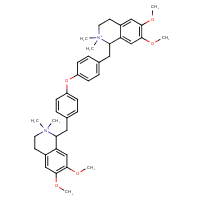Structure InformationCompound Identification

SMILES

COC1=C(OC)C=C2C(CC3=CC=C(OC4=CC=C(CC5C6=CC(OC)=C(OC)C=C6CC[N+]5(C)C)C=C4)C=C3)[N+](C)(C)CCC2=C1

InChIKey

InChIKey=BRLVMMPAGUOHLZ-UHFFFAOYSA-N

Formula

C40H50N2O5

Mass

638.848